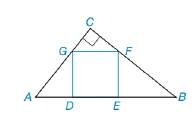Chapter 8.1, Problem 53E### Elementary Geometry for College St...

6th Edition
Daniel C. Alexander + 1 other
ISBN: 9781285195698

#### Solutions

Chapter
Section### Elementary Geometry for College St...

6th Edition
Daniel C. Alexander + 1 other
ISBN: 9781285195698
Textbook Problem
1 views

# Square DEFG is inscribed in right △ A B C , as shown. If A D = 6 and E B = 8 , find the area of square DEFG.To determine

To find:

The area of the square DEFG.

Explanation

Finding the area of the square is followed by the geometric formula,

Area of the square A=s2 unit2,

Where s is the length of the each side.

Calculation:

Given:

Square DEFG is inscribed in right ABC, as shown below.

Let x be the length of each side of the equare.

By the triangle ABC,

Let x be the angle of LB and θ be the angle of LA.

(i.e.) LA=LF and LB=LG

By the figure,

tanθ=8x=x6

(i

### Still sussing out bartleby?

Check out a sample textbook solution.

See a sample solution

#### The Solution to Your Study Problems

Bartleby provides explanations to thousands of textbook problems written by our experts, many with advanced degrees!

Get Started

#### Expand each expression in Exercises 122. (x+yxy)2

Finite Mathematics and Applied Calculus (MindTap Course List)

#### Define sampling with replacement and explain why is it used?

Statistics for The Behavioral Sciences (MindTap Course List)

#### If limx0sinxx=1, the angle x must be measured in: a) radians b) degrees c) it does not matter

Study Guide for Stewart's Single Variable Calculus: Early Transcendentals, 8th

#### Self Check 5 Solve: |3x63|+212

College Algebra (MindTap Course List)